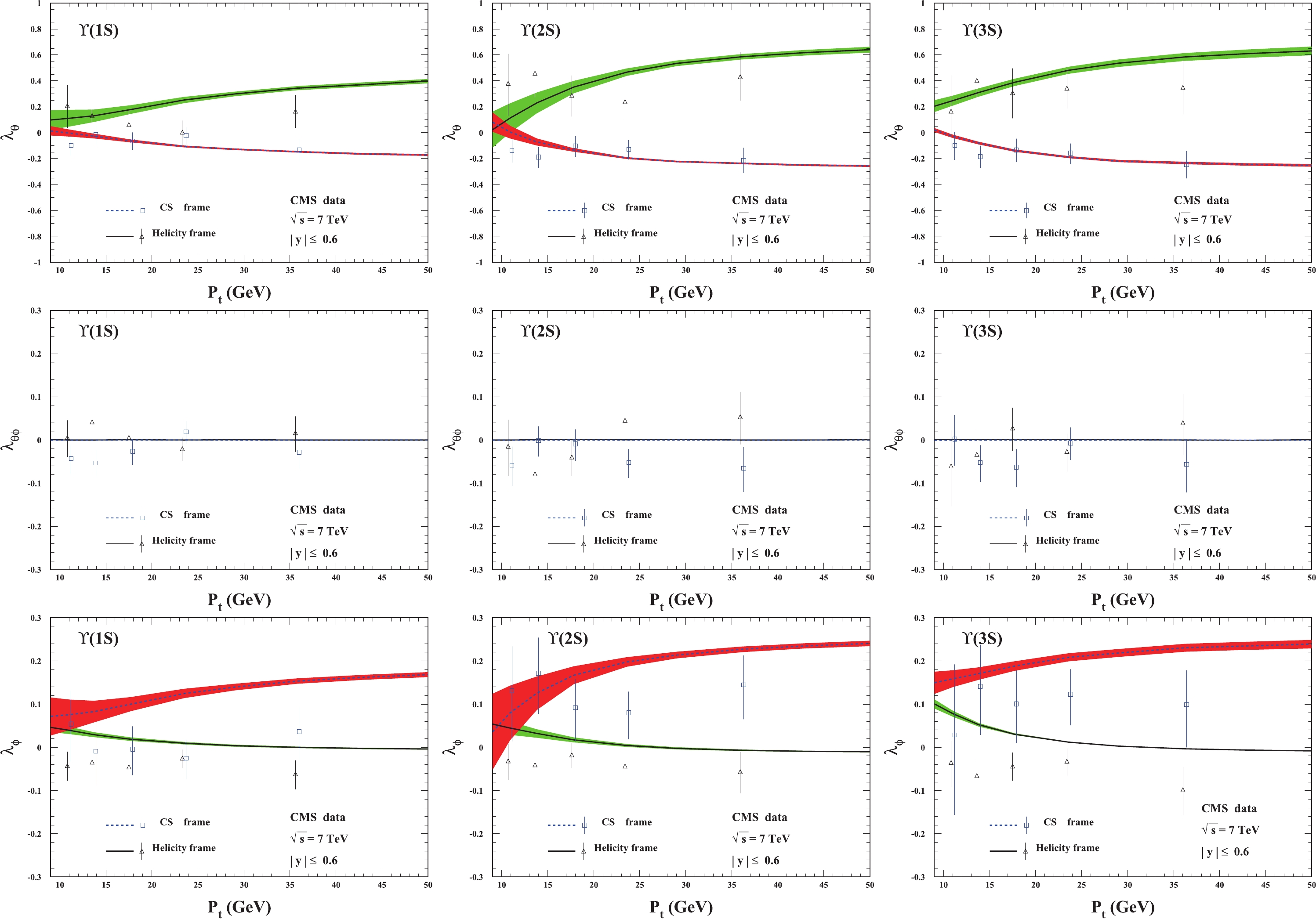# Complete study on polarization of ${\Upsilon {(nS)}}$hadroproduction at QCD next-to-leading order• By applying the nonrelativistic quantum chromodynamics factorization formalism to $\Upsilon(1S,2S,3S)$hadroproduction, a complete analysis of the polarization parameters $\lambda_{\theta}$, $\lambda_{\theta\phi}$, and $\lambda_{\phi}$for the production is presented at QCD next-to-leading order. With the long-distance matrix elements extracted from experimental data for the production rate and polarization parameter $\lambda_{\theta}$of $\Upsilon$hadroproduction, our results provide a good description of the measured parameters $\lambda_{\theta\phi}$and $\lambda_{\phi}$in both the helicity and Collins-Soper frames. In our calculations, the frame invariant parameter $\tilde{\lambda}$is consistent in the two frames. Finally, we mention that there are discrepancies between the available experimental data and corresponding theoretical predictions for $\tilde{\lambda}$.
••Get Citation
Yu Feng, Bin Gong, Chao-Hsi Chang and Jian-Xiong Wang. The complete study on polarization of ${\Upsilon }$(nS) hadroproduction at QCD next-to-leading order[J]. Chinese Physics C. doi: 10.1088/1674-1137/abc682
Yu Feng, Bin Gong, Chao-Hsi Chang and Jian-Xiong Wang. The complete study on polarization of ${\Upsilon }$(nS) hadroproduction at QCD next-to-leading order[J]. Chinese Physics C.Milestone
Received: 2020-09-08
Article Metric

Article Views(307)
PDF Downloads(31)
Cited by(0)
Policy on re-use
To reuse of Open Access content published by CPC, for content published under the terms of the Creative Commons Attribution 3.0 license (“CC CY”), the users don’t need to request permission to copy, distribute and display the final published version of the article and to create derivative works, subject to appropriate attribution.
###### 通讯作者: 陈斌, bchen63@163.com
• 1.

沈阳化工大学材料科学与工程学院 沈阳 110142

Title:
Email:

## Complete study on polarization of ${\Upsilon {(nS)}}$hadroproduction at QCD next-to-leading order

###### Corresponding author: Jian-Xiong Wang, jxwang@ihep.ac.cn
• 1. Department of Physics, College of Basic Medical Sciences, Army Medical University, Chongqing 400038, China
• 2. CAS Key Laboratory of Theoretical Physics, Institute of Theoretical Physics, Chinese Academy of Sciences, Beijing 100190, China
• 3. Institute of High Energy Physics, Chinese Academy of Sciences, P.O.Box 918(4), Beijing 100049, China
• 4. School of Physical Sciences, University of Chinese Academy of Sciences, Beijing 100190, China

Abstract: By applying the nonrelativistic quantum chromodynamics factorization formalism to $\Upsilon(1S,2S,3S)$hadroproduction, a complete analysis of the polarization parameters $\lambda_{\theta}$, $\lambda_{\theta\phi}$, and $\lambda_{\phi}$for the production is presented at QCD next-to-leading order. With the long-distance matrix elements extracted from experimental data for the production rate and polarization parameter $\lambda_{\theta}$of $\Upsilon$hadroproduction, our results provide a good description of the measured parameters $\lambda_{\theta\phi}$and $\lambda_{\phi}$in both the helicity and Collins-Soper frames. In our calculations, the frame invariant parameter $\tilde{\lambda}$is consistent in the two frames. Finally, we mention that there are discrepancies between the available experimental data and corresponding theoretical predictions for $\tilde{\lambda}$.

### HTMLI.   INTRODUCTIONII.   THEORY DESCRIPTIONIII.   NUMERICAL RESULTS
Reference (37)
PDF查看关注分享

Top

### 目录/DownLoad:  Full-Size Img  PowerPoint